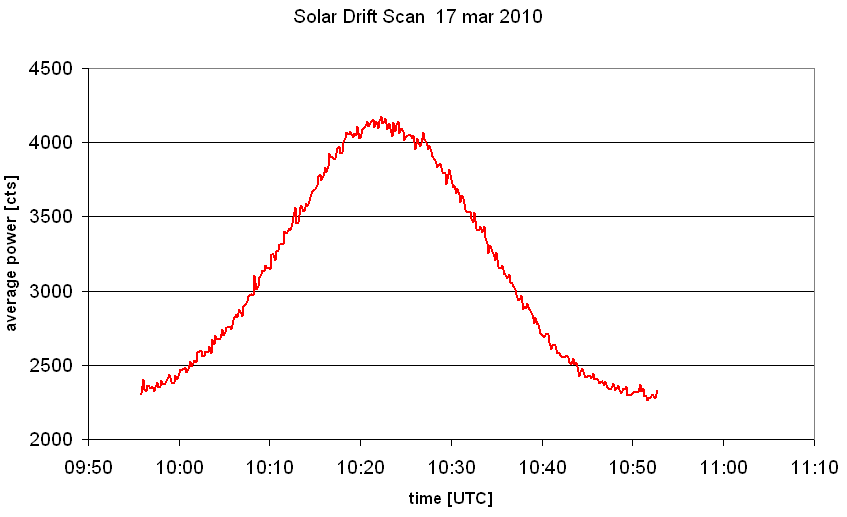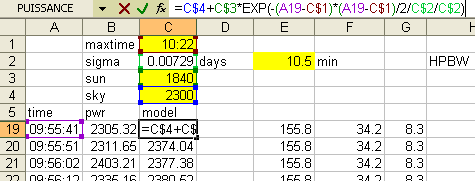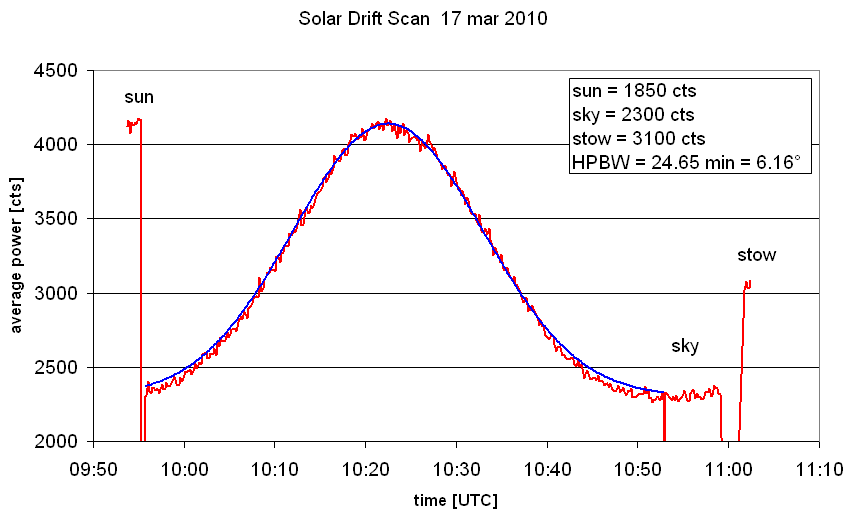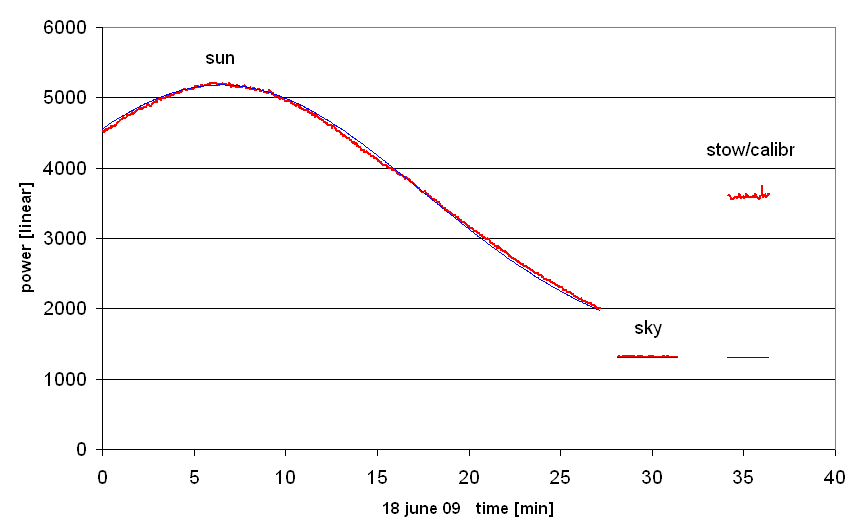Solar Observations with ESA-Haystack

Joachim Köppen Strasbourg 2010

The other aspect is about the telescope: Since the empty sky provides radio wave emission only at negligible intensity, the signal that we measure is essentially the background noise from our receiver. Comparing the solar signal with that of the sky we obtain a measure of the sensitivity of our instrument: The larger the ratio of the solar and sky signals is, more sensitive is the system. We express this more quantitatively as the System Temperature.

The third aspect is also technical: We can determine the width of the antenna pattern, i.e. the Half-Power Beam Width (HPBW) which describes also the angular resolution possible with the antenna. This is done by letting the Sun - which we use as a source with a constant signal - pass through the antenna beam, and measure the time it takes. With the knowledge of the Earth rotation's angular velocity we convert the time interval into an angle, the HPBW.

Observational procedure

After starting up the system, we recommend to follow this sequence:

Analysis

Let's suppose that we use Microsoft Excel to do the interpretation of the data. Then we recommend to follow this sequence:

• To import your text file go to: File -> Import-> Text File -> Select your Text File -> Delimited Text file -> Delimiter is spaces -> Finish
• Each row represents a single spectrum taken by the telescope at a certain time instant. The columns from left to right represent: Time, Azimuth, Elevation, offsets in azimuth and elevation, velocity with respect to the Local Standard of Rest (LSR), the first frequency in the spectrum, the frequency increment, an integer number indicating the frequency mode, the number of frequency points in the spectrum, followed by the flux at the first frequency all though to the flux at the last frequency.
• We shall need only the times and the fluxes
• Insert a new column right after the first column. Fill the cells with the AVERAGE of the flux values in this row. However, leave out the first eight and the last eight cells. Do this for all the rows in your data.
• Now make a scatter plot of the first two columns, which gives the average power as a function of time. After some cosmetics it may look like this:• From this plot, we can determine the maximum signal of the Sun (here: F_sun = 4150 cts), the background level of the empty sky (F_sky = 2300 cts), and we have the measurement at stow from the emission of the library wall calibrator (F_cal = 3100). These numbers allow us to compute:
• the true signal of the calibrator: F_cal-F_sky = 3100-2300 = 800 cts which corresponds to the calibrator's temperature of about 290 K
• the true signal of the Sun: F_sun-F_sky = 4150-2300 = 1850 cts, from which we compute the Sun's antenna temperature as
T_ant(Sun) = (F_sun-F_sky)/(F_cal-F_sky)*T_cal = 1850/800*290 = 670 K
• Next, we look at which times the signal was halfway between the maximum and the sky, that is where its value was (4150+2300)/2 = 3225 cts. Here, the result will be: 10:10:34 and 10:34:38. This makes 24 minutes and 04 seconds for the time the Sun passed through the antenna beam, at its half-power points.
• The Earth revolves once in 24 hours, or 15°/hr or 0.25°/min. Thus, the 24 minutes give 6° as the Half-Power Beam Width of the antenna. A more accurate value is obtained, if we take into account that depending on its actual declination d the Sun moves with somewhat slower speed of 0.25°/min*cos(d). The declination of the Sun you may obtain from this tool. In our example of data taken close to the spring equinox this correction is minute.
• With the antenna's HPBW known, we now can derive the temperature on the surface of the Sun: Since the Sun has an angular diameter of about 0.5°, much smaller than the antenna beam, its temperature must be correspondingly hotter than the library wall which fills the entire antenna beam. Thus the true solar temperature is
T_sun = T_ant(Sun) * (HPBW/0.5))˛ = 96500 K
You may be surprised about such a high value ... but this is the temperature at the bottom of the corona, which is the outer layer of the Sun which we observe at 1.4 GHz! If we could observe at even lower frequencies, we would get values up to a few million K!
• Finally, we can derive the system temperature of the telescope, from the formula
T_sys = T_cal / (F_cal/F_sky - 1) = 290/(3100/2300 - 1) = 833 K
This rather high temperature indicates that our receiving system is not yet optimal, as one should expect for this telescope about 200 K or a bit less.

You might wish to do a more sophisticated analysis by fitting a simple model to the data:

• We assume that the signal is composed of a Gaussian function representing the antenna pattern and a constant background:
F(t) = F_sky + S_sun * exp( -0.5((t-tmax)/s)˛ )
with the free parameters
• F_sky ... the level of the background
• S_sun ... the true level of the sun's radiation
• tmax ... the time of the maximum power
• s the width of the Gaussian in time units. Note that in Excel time is measured in days, so that
s = (HPBW/2.3548)/(360° * cosd)
in all beauty
• Insert a new column after column B, and also add a couple of rows into which you can place the cells that will old the free parameters. Fill the new column with the predicted flux levels, as shown below:You see that I marked the cells where I can input a value with a colour.
• Make a plot of the observed and predicted fluxes as functions of time, or simply add the model curve to your existing graph. Now adjust all the parameters until you get a best match of the model curve with your observations, such as shown below:This plot shows also some measurements before and after the drift scan, and it collects the best parameter values in a small text table.
• The plot shows quite clearly that the assumption of a Gaussian profile for the antenna pattern is not quite perfect. The sensitivity of the main lobe drops a bit faster than the Gaussian. Also, it would have been better to execute a bit more patience after the drift scan to let the curve go really flat ... but my excuse is that at that time i moved the telescope 90° off to the East, to get the sky really far away from the Sun ;-)

Our results

Our first solar observation was done on 18 june 2009, the day of First Light:This was done as decribed above. At this time, we operated the receiver without any bandpass filter, because we did not yet suffer from strong interference. Therfore, the results were much better:
• the level of the background: 1317 cts
• the height of the maximum solar signal (background subtracted): 5180-1317 = 3863 cts
• the level corresponding to 290K: 3599-1317 = 2282 cts
• the antenna temperature of the Sun's peak signal: 290K * 3863/2282 = 491 K
• by fitting a gaussian curve (the thin blue curve) to the solar data, we determine its width s = 11.04 minutes of time. As the Sun was at declination d = +23.40°, it moved across the sky with angular speed of 0.25°/min*cos(d). Taken all together, this gives a HPBW of our antenna of 5.95°.
• deviations of the measured curve from gaussian curve are slight, but significant!
For that day, we also can use the Solar radio data from NOAA. They show that the solar flux was very constant throughout the day, and the flux measured at noon UTC by the observatory on San Vito Island (SVI) at 1415 MHz was 54 SFU (solar flux units ... 1 SFU = 10000 Jy). Our measured solar antenna temperature of 490 K would be consistent with an antenna efficiency of 60 percent (see System Temperature for more details).

Now that we have installed a narrow band-pass filter to protect the receiver from interference caused by transmitters operating on frequencies other than 1420 MHz, the unavoidable losses in the filter brought up our present system temperature to about 1000 K. This means that we need to do some more work to optimize the system!

last update: Apr. 2010 J.Köppen